Next: Magnetic Field of a Up: Magnetism Previous: The Hall Effect

## Ampère's Circuital Law

Consider a long thin wire carrying a steady current. Suppose that the wire is orientated such that the current flows along the-axis. Consider some closed loop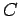in the-plane which circles the wire in an anti-clockwise direction, looking down the-axis. Suppose thatis a short straight-line element of this loop. Let us form the dot product of this element with the local magnetic field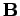. Thus,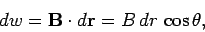(173)

whereis the angle subtended between the direction of the line element and the direction of the local magnetic field. We can calculate a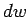for every line element which makes up the loop. If we sum all of thevalues thus obtained, and take the limit as the number of elements goes to infinity, we obtain the line integral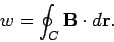(174)

What is the value of this integral? In general, this is a difficult question to answer. However, let us consider a special case. Suppose thatis a circle of radiuscentred on the wire. In this case, the magnetic field-strength is the same at all points on the loop. In fact,(175)

Moreover, the field is everywhere parallel to the line elements which make up the loop. Thus,(176)

or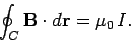(177)

In other words, the line integral of the magnetic field around some circular loop, centred on a current carrying wire, and in the plane perpendicular to the wire, is equal to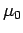times the current flowing in the wire. Note that this answer is independent of the radiusof the loop: i.e., the same result is obtained by taking the line integral around any circular loop centred on the wire.

In 1826, Ampère demonstrated that Eq. (177) holds for any closed loop which circles around any distribution of currents. Thus, Ampère's circuital law can be written:

The line integral of the magnetic field around some closed loop is equal to thetimes the algebraic sum of the currents which pass through the loop.
In forming the algebraic sum of the currents passing through the loop, those currents which the loop circles in an anti-clockwise direction (looking against the direction of the current) count as positive currents, whereas those which the loop circles in a clockwise direction (looking against the direction of the current) count as negative currents.

Ampère's circuital law is to magnetostatics (the study of the magnetic fields generated by steady currents) what Gauss' law is to electrostatics (the study of the electric fields generated by stationary charges). Like Gauss' law, Ampère's circuital law is particularly useful in situations which possess a high degree of symmetry.Next: Magnetic Field of a Up: Magnetism Previous: The Hall Effect
Richard Fitzpatrick 2007-07-14